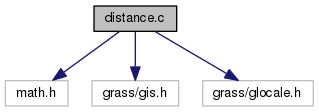GRASS Programmer's Manual  6.5.svn(2014)-r66266
gis/distance.c File Reference
`#include <math.h>`
`#include <grass/gis.h>`
`#include <grass/glocale.h>`
Include dependency graph for gis/distance.c:Go to the source code of this file.

## Functions

int G_begin_distance_calculations (void)
Begin distance calculations. More...

double G_distance (double e1, double n1, double e2, double n2)
Returns distance in meters. More...

double G_distance_between_line_segments (double ax1, double ay1, double ax2, double ay2, double bx1, double by1, double bx2, double by2)
Returns distance between two line segments in meters. More...

double G_distance_point_to_line_segment (double xp, double yp, double x1, double y1, double x2, double y2)
Returns distance between a point and line segment in meters. More...

## Function Documentation

 int G_begin_distance_calculations ( void )

Begin distance calculations.

Initializes the distance calculations. It is used both for the planimetric and latitude-longitude projections.

Returns
0 if projection has no metrix (ie. imagery)
1 if projection is planimetric
2 if projection is latitude-longitude

Definition at line 44 of file gis/distance.c.

Referenced by Gs_distance(), and Vect_line_geodesic_length().

 double G_distance ( double e1, double n1, double e2, double n2 )

Returns distance in meters.

This routine computes the distance, in meters, from x1,y1 to x2,y2. If the projection is latitude-longitude, this distance is measured along the geodesic. Two routines perform geodesic distance calculations.

Parameters
 [in] e1,n1 east-north coordinates of first point [in] e2,n2 east-north coordinates of second point
Returns
distance

Definition at line 78 of file gis/distance.c.

References G_geodesic_distance().

Referenced by G_distance_point_to_line_segment(), and Gs_distance().

 double G_distance_between_line_segments ( double ax1, double ay1, double ax2, double ay2, double bx1, double by1, double bx2, double by2 )

Returns distance between two line segments in meters.

Parameters
 [in] ax1,ay2,ax2,ay2 first segment [in] bx1,by2,bx2,by2 second segment
Returns
double

Definition at line 96 of file gis/distance.c.

References G_distance_point_to_line_segment(), G_intersect_line_segments(), and y.

 double G_distance_point_to_line_segment ( double xp, double yp, double x1, double y1, double x2, double y2 )

Returns distance between a point and line segment in meters.

Parameters
 [in] xp,yp point coordinates [in] x1,x1 segment point coordinates [in] x2,y2 segment point coordinates
Returns
distance

Definition at line 126 of file gis/distance.c.

References G_distance(), G_intersect_line_segments(), G_warning(), and y.

Referenced by G_distance_between_line_segments().